# Latin Squares

##### Age 11 to 18

Published 2002 Revised 2011

A Latin square of order n is an array of n symbols in which each symbol occurs exactly once in each row and exactly once in each column. See the interactivity Teddy Town for some examples of Latin squares.

Construct some Latin squares for yourself and see how many different arrangements you can find for each value of n. Two Latin squares are essentially the same, the mathematical term is isomorphic, if one can be transformed into the other by re-naming the elements or by interchanging rows or interchanging columns.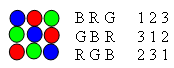For example the coloured discs in this illustration form a Latin square of order 3. This Latin square is isomorphic to the square with the symbols B, R and G and to the square with symbols 1, 2 and 3.

An interactivity can be found in http://www.cut-the-knot.org/arithmetic/latin.shtml .

Latin squares of all orders $m> 2$ can be constructed using modular arithmetic as in this example for $m=5$. The entry $S_{i,j}$ in row $i$ column $j$ is given by $S_{i,j}=i+j$ (mod $5$), where this is defined to be the remainder when the sum $i+j$ is divided by $5$.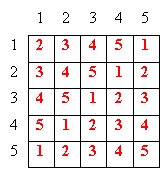For example the entry in the 4th row and 3rd column is given by $S_{4,3}=4+3$ (mod $5$) $=7$ (mod $5$) $=2$.

The same arrays can be found by simply cycling the elements so this method becomes more useful when solving problems involving combinations of two Latin squares.

Two Latin squares are said to be orthogonal if they can be combined cell by cell so that each cell consists of a different pair of symbols.

 The two Latin squares of order 3, given in paragraph three above are not orthogonal because in the first row and first column the combination is B1 and the same combination occurs in the second row and second column. However the Latin squares on the right are orthogonal.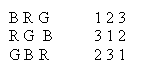You can construct orthogonal Latin squares $S_{i,j}$ and $T_{i,j}$ of prime order $m$ where the $S_{i,j}=s i+j$ (mod $m$) and $T_{i,j}=t i+j$ (mod $m$) and $s$ is not equal to $t$. For example here are orthogonal Latin squares of order $7$ taking $s=1$ and $t=2$.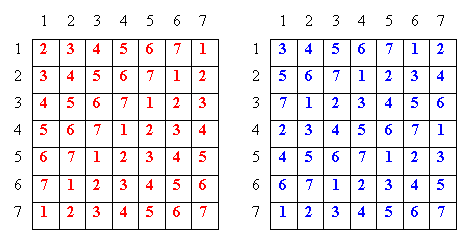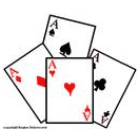Try making orthogonal Latin squares for yourself using the honour cards (Ace, King, Queen, Jack) from a standard pack of playing cards. First ignore the suits altogether and concentrate on arranging the 4 by 4 array so that each row and column has an Ace, a King, a Queen and a Jack in it, or equivalently so that no row or column has two Aces, or two Kings etc. Note down the arrangement. Now concentrate on the suits and ignore the pictures and note down the arrangement of the suits. Because each card is different, that is all the combinations are different, for example Ace of Spades, Ace of Hearts, Ace of Diamonds and Ace of Clubs, the Latin square of 'suits' is necessarily orthogonal to the Latin square of 'pictures'. In 1783 Euler made a conjecture about orthogonal Latin squares and it took nearly 200 years for mathematicians to prove that orthogonal Latin squares exist for all orders except 2 and 6. How many different solutions can you find to the following problem that was originally posed by Euler? "Arrange 25 officers, each having one of five different ranks and belonging to one of five different regiments, in a square formation 5 by 5, so that each row and each file contains just one officer of each rank and just one from each regiment." The corresponding problem with 36 officers of six different ranks and belonging to six different regiments cannot be solved although for 49 officers it is easy to solve as shown above.